Home > CC4 > Chapter 7 > Lesson 7.1.4 > Problem7-46

7-46.
1. For each pair of triangles below, determine if the triangles are congruent. If the triangles are congruent, Homework Help ✎

• Complete the congruence statement

• State the congruence property

• Determine a sequence of rigid transformations that maps one triangle onto the other

2. Otherwise, explain why you cannot conclude that the triangles are congruent. Note that the figures are not necessarily drawn to scale.

1. ΔABC ≅ Δ _____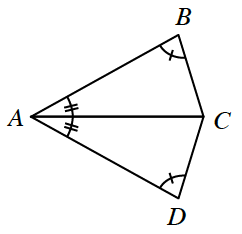2. ΔPLM ≅ Δ _____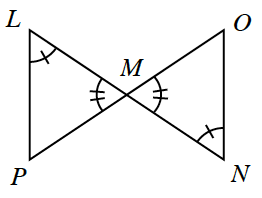3. ΔWXY ≅ Δ _____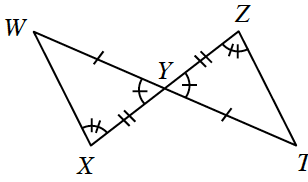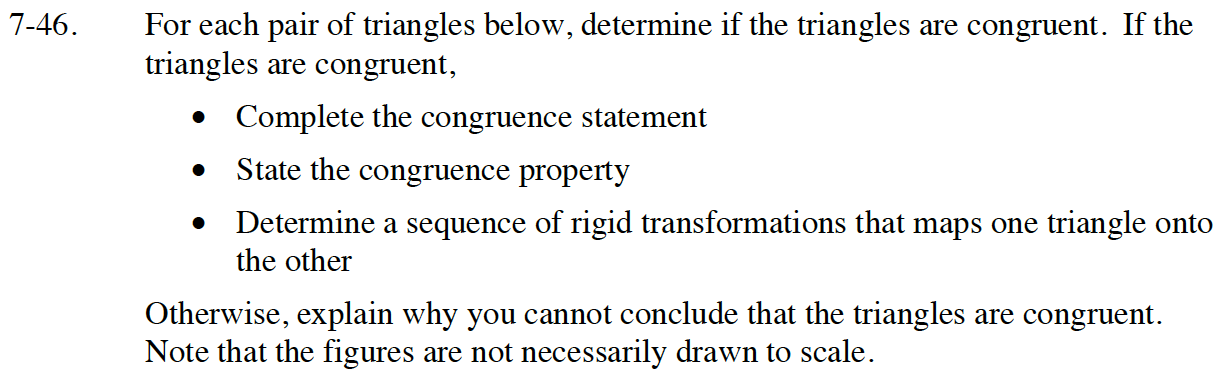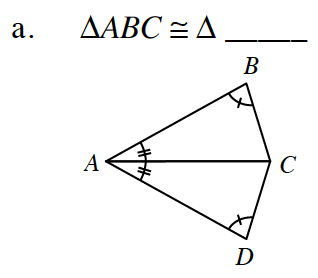ADC; AAS ≅ (Remember AC=CA by reflexive).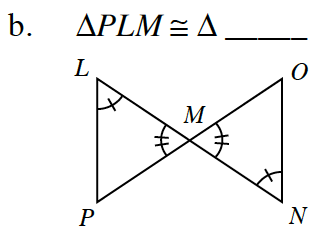No solution; only the angles are congruent. These triangles can only be proved to be similar by AA∼ but not congruent.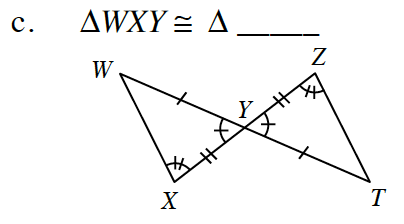TZY; SAS ≅ or AAS≅.